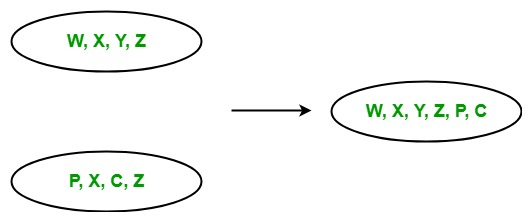Related Articles
LINQ | Set Operator | Union
• Last Updated : 22 May, 2019

In LINQ, Set operators are those operators in query expression which return a result set which is based on the existence or non-existence of the equivalent elements within the same or different collections or sequences or sets. The standard query operator contains the following set operators:

1. Union
2. Intersect
3. Except
4. Distinct

#### Union

The Union operator returns the set union. Or in other words, we can say that it returns the set which contains the unique elements that appear in the given input collections, or sequence.• It does not support query syntax in C# and VB.Net languages. But you can use Union method on query variable or you can wrap your query in brackets and then the call Union method.
• It support method syntax in both C# and VB.Net languages.
• It present in both the Queryable and Enumerable class.
• It is implemented by using deferred execution.
• When you are working with the collections of complex types you must use IEqualityComparer interface, otherwise, the Union method will give you incorrect result.

Example 1:

 `// C# program to find the union ``// of the given sequence``using` `System;``using` `System.Linq;`` ` `class` `GFG {`` ` `    ``static` `public` `void` `Main()``    ``{`` ` `        ``// Data source``        ``char``[] sequence1 = {``'m'``, ``'q'``, ``'o'``, ``'s'``, ``'y'``, ``'a'``};``        ``char``[] sequence2 = {``'p'``, ``'t'``, ``'r'``, ``'s'``, ``'w'``, ``'z'``};`` ` `        ``// Display the sequences``        ``Console.WriteLine(``"Sequence 1 is: "``);`` ` `        ``foreach``(``var` `s1 ``in` `sequence1)``        ``{``            ``Console.WriteLine(s1);``        ``}`` ` `        ``Console.WriteLine(``"Sequence 2 is: "``);`` ` `        ``foreach``(``var` `s2 ``in` `sequence2)``        ``{``            ``Console.WriteLine(s2);``        ``}`` ` `        ``// Get union of the given sequence``        ``// Using Union function``        ``var` `result = sequence1.Union(sequence2);`` ` `        ``Console.WriteLine(``"Unique Sequence: "``);`` ` `        ``foreach``(``var` `val ``in` `result)``        ``{``            ``Console.WriteLine(val);``        ``}``    ``}``}`
Output:

```Sequence 1 is:
m
q
o
s
y
a
Sequence 2 is:
p
t
r
s
w
z
Unique Sequence:
m
q
o
s
y
a
p
t
r
w
z
```

Example 2:

 `// C# program to find the common ``// languages known by the employees``using` `System;``using` `System.Linq;``using` `System.Collections.Generic;`` ` `// Employee details``public` `class` `Employee1 {`` ` `    ``public` `int` `emp_id1``    ``{``        ``get``;``        ``set``;``    ``}`` ` `    ``public` `string` `emp_name1``    ``{``        ``get``;``        ``set``;``    ``}``    ``public` `string` `emp_lang1``    ``{``        ``get``;``        ``set``;``    ``}``}``// Employee details``public` `class` `Employee2 {`` ` `    ``public` `int` `emp_id2``    ``{``        ``get``;``        ``set``;``    ``}`` ` `    ``public` `string` `emp_name2``    ``{``        ``get``;``        ``set``;``    ``}``    ``public` `string` `emp_lang2``    ``{``        ``get``;``        ``set``;``    ``}``}``public` `class` `GFG {`` ` `    ``// Main method``    ``static` `public` `void` `Main()``    ``{``        ``List emp1 = ``new` `List() {`` ` `            ``new` `Employee1() {emp_id1 = 209, emp_name1 = ``"Anjita"``,``                                               ``emp_lang1 = ``"C#"``},`` ` `            ``new` `Employee1() {emp_id1 = 210, emp_name1 = ``"Soniya"``,``                                                ``emp_lang1 = ``"C"``},`` ` `            ``new` `Employee1() {emp_id1 = 211, emp_name1 = ``"Rohit"``,``                                            ``emp_lang1 = ``"Java"``},`` ` `        ``};`` ` `        ``List emp2 = ``new` `List() {`` ` `            ``new` `Employee2() {emp_id2 = 290, emp_name2 = ``"Anjita"``,``                                               ``emp_lang2 = ``"C#"``},`` ` `            ``new` `Employee2() {emp_id2 = 212, emp_name2 = ``"MaMa"``, ``                                         ``emp_lang2 = ``"Python"``},`` ` `            ``new` `Employee2() {emp_id2 = 233, emp_name2 = ``"Rima"``, ``                                           ``emp_lang2 = ``"Java"``},`` ` `        ``};`` ` `        ``// Query to find the common languages``        ``// known by the employees``        ``// Using Union method``        ``var` `res = emp1.Select(e => e.emp_lang1).Union(emp2.Select(e => e.emp_lang2));``         ` `        ``Console.WriteLine(``"Common Languages: "``);``         ` `        ``foreach``(``var` `val ``in` `res)``        ``{``            ``Console.WriteLine(val);``        ``}``    ``}``}`
Output:
```Common Languages:
C#
C
Java
Python
```

Attention reader! Don’t stop learning now. Get hold of all the important DSA concepts with the DSA Self Paced Course at a student-friendly price and become industry ready.

My Personal Notes arrow_drop_up